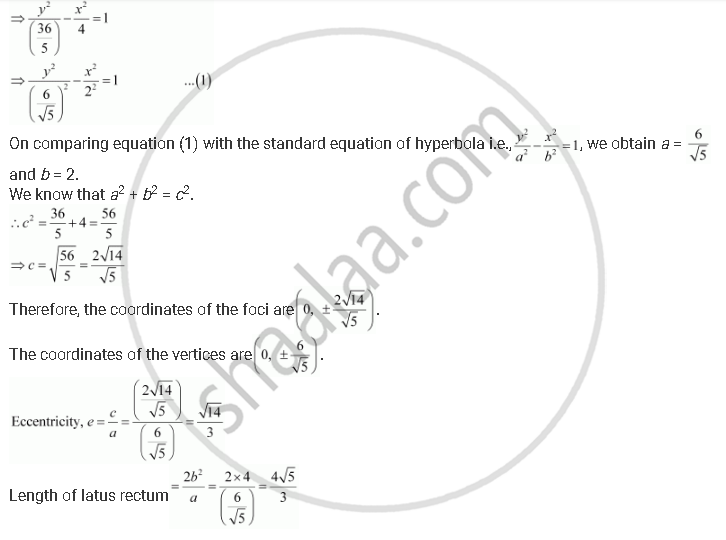CBSE (Arts) Class 11CBSE
Share
Notifications

View all notifications
Books Shortlist
Your shortlist is empty

# Find the Coordinates of the Foci and the Vertices, the Eccentricity, and the Length of the Latus Rectum of the Hyperbola 5y2 – 9x2 = 36 - CBSE (Arts) Class 11 - Mathematics

Login
Create free account

Forgot password?
ConceptHyperbola Eccentricity

#### Question

Find the coordinates of the foci and the vertices, the eccentricity, and the length of the latus rectum of the hyperbola 5y2 – 9x2 = 36

#### Solution

The given equation is 5y2 – 9x2 = 36.Is there an error in this question or solution?

#### APPEARS IN

Solution Find the Coordinates of the Foci and the Vertices, the Eccentricity, and the Length of the Latus Rectum of the Hyperbola 5y2 – 9x2 = 36 Concept: Hyperbola - Eccentricity.
S